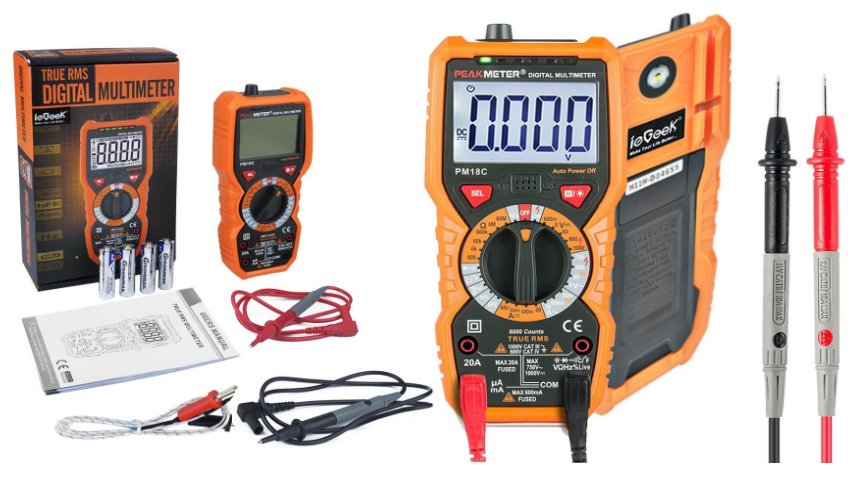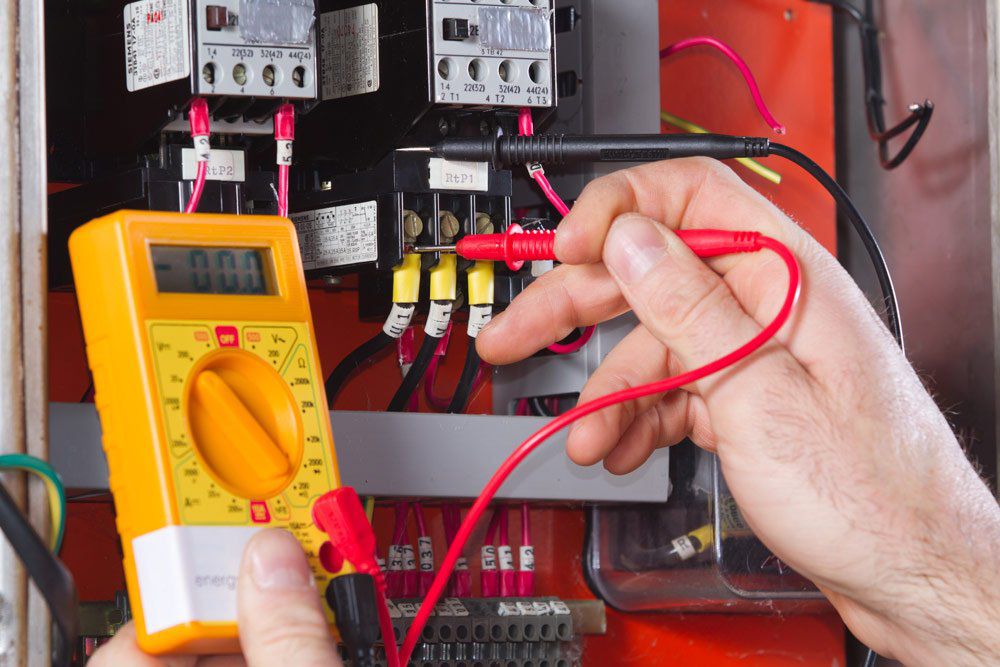# How To Measure Current Flow Using Ammeter

0
291

According to the definition on the internet, a current is a flow of electrical charge carriers, usually electrons or electron-deficient atoms. Capital letter ‘I’ is used as a universal symbol for current with a standard unit in ampere, which is symbolized by A. To measure current in a circuit, you can use an Ammeter.

### What Is An Ammeter?The name ammeter was derived from combining the words ampere and meter because it is an instrument used for measuring the strength of an electric current in terms of amperes. In some cases, the Ammeter Shunts are built inside the instrument while in others it is externally connected to the circuit. The most common way in measuring current in a circuit is to break the circuit open and insert an ammeter in series or in-line with the circuit, in such a way, all electron flowing through the circuit also have to get pass the meter. If you set the ammeter up in parallel, it will create a short circuit and will not measure the current correctly.

### Proper Way To Use An AmmeterIn measuring current using an ammeter, you must first set the range of your ammeter. Begin by choosing the highest range for current to prevent the internal fuse from blowing up. Then shift the selector switch to lower range position until the most reliable indicator is acquired on the meter display. Depending on your needs, set the current type to DC or AC.

The next step is to connect the terminals of the ammeter in series of the resistance or load. Through this, the ammeter will have the equivalent amount of current flowing in the circuit. For a clear understanding, let us take the example of a simple circuit. Imagine a bulb connected to a battery. Try to remove any one of the terminals of the bulb in such a way that one probe of the ammeter is connected to the lamp and the other probe is connected to the battery. From there, you can already read on the ammeter the amount of the current flowing in your circuit. After you determine how much current is present, you can already disconnect the ammeter and put the circuit back to its original set up.

However, there is a warning when you measure the electric current. Avoid connecting the ammeter’s probes straight to the battery because this will induce a short circuit in the ammeter. If you desire to know the flow of a battery, it is required to attach a resistance across the battery and then connect an ammeter series for an accurate reading.

Two other methods are widely used in measuring current aside from using an ammeter such as magnetic method which is capable of measuring large currents. Another way is by using a galvanometer. It is a device which is only used to detect the presence of current in a circuit. What is the difference between ammeter and galvanometer? A galvanometer shows both the direction and the magnitude of the current, while the ammeter only shows the magnitude of the current.

Regardless of the method, you want to use; current measurements need to be done to find out whether a circuit is operating correctly, or to discover other facts associated with its current consumption.